隐马尔可夫模型

原理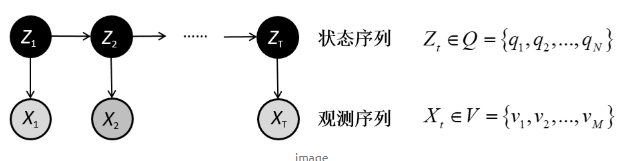（1）概率计算问题：给定模型$\lambda=(A,B,\Pi)$和观测序列$O=(o_1,o_2,\ldots,o_T)$，计算在模型$\lambda$下观测序列O出现的概率$P(O|\lambda)$。

（2）学习问题：已知观测序列$O=(o_1,o_2,\ldots,o_T)$，估计模型$\lambda=(A,B,\Pi)$参数，使得在该模型下观测序列概率$P(O|\lambda)$最大。即用极大似然估计的方法估计参数。

（3）预测问题（解码问题）：已知模型$\lambda=(A,B,\Pi)$和观测序列$O=(o_1,o_2,\ldots,o_T)$，求对给定观测序列条件概率$P(I|O)$最大的状态序列$I=(i_1,i_2,\ldots,i_T)$。即给定观测序列，求最优可能的对应的状态序列。

概率计算问题

前向算法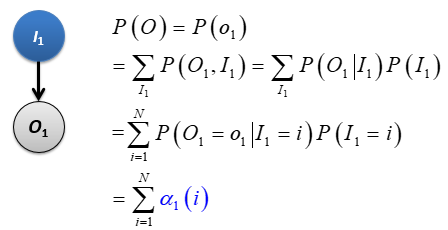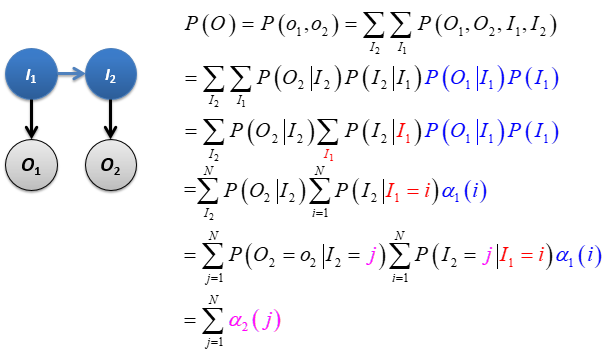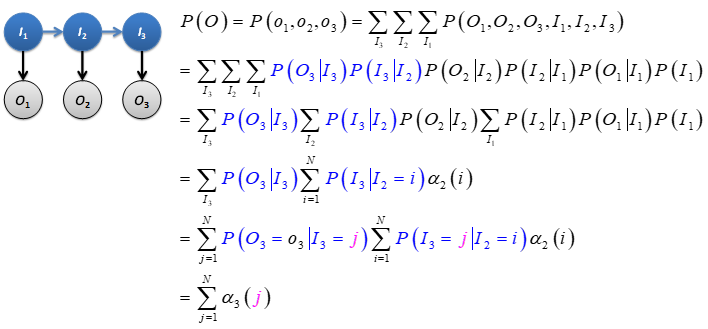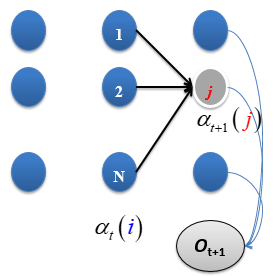（1）初始值

（2）递推，$t=1,2,\ldots,T-1$

（3）终止

后向算法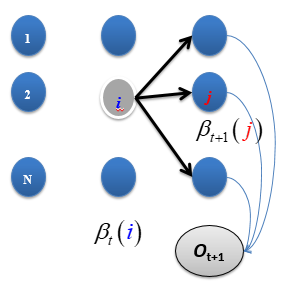（1）初始值

（2）递推，$t=T-1,T-2,\dots,1$

（3）终止

预测问题（解码问题）

近似算法

• 不能保证预测的状态序列是整体最优的；
• 状态序列中可能存在转移概率为0的相邻状态。

维特比算法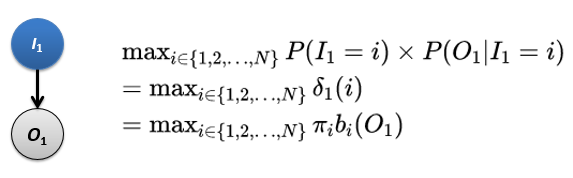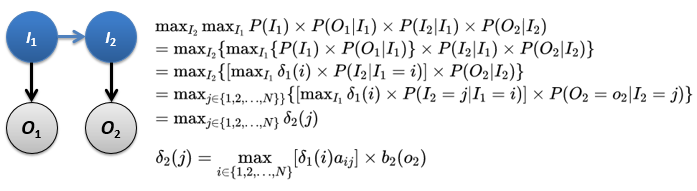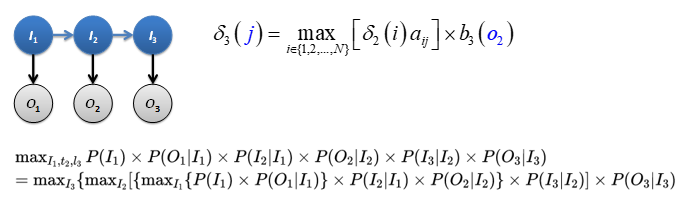（1）初始值

（2）递推，对$t=2,\ldots,T$

（3）终止

（4）最优路径回溯，对$t=T-1,T-2,\ldots,1$